## Problem Solving Lesson 35 Convergence of three perpendicular bisectors and three altitudes in a triangle (Chapter 9 Math 7 Connecting) – Math Book

Problem Solving Lesson 35 Convergence of three perpendiculars and three altitudes in a triangle (Chapter 9 Math 7 Connecting)
==========

### Solve problem 9.26 page 81 Math 7 Textbook Connecting knowledge volume 2 – KNTT

Let H be the orthocenter of a non-square triangle ABC. Find the orthocenter of the triangles HBC, HCA, HAB

Detailed instructions for solving problem 9.26

Solution method

AH ⊥ BC at N, BH ⊥ AC at P, CH ⊥ AB at M => C is the orthocenter of triangle AHB.

Prove that B is orthocenter of HAC, A is orthocenter of triangle HBC

Detailed explanation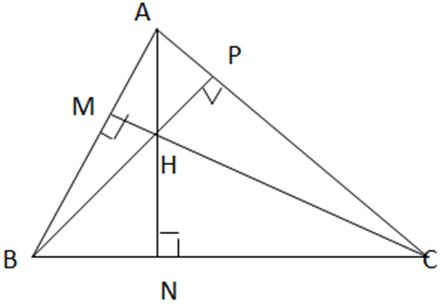In ΔABC we have H as the orthocenter, so:

AH BC at N, BH AC at P, CH AB at US

In ΔAHB, we have:

AC BH

BC AH

=> C is the orthocenter of triangle AHB.

In ΔHAC, we have:

AB ONLY

CB AH

=> B is the orthocenter of ΔHAC.

In ΔHBC, we have:

THREE HC

CA BH

=> A is the orthocenter of triangle HBC

–>

— *****

### Solve problem 9.27 page 81 Math textbook 7 Connecting knowledge volume 2 – KNTT

Let ABC be a triangle with \(\widehat{A}\) = 100° and orthocenter H. Find angle BHC

Detailed instructions for solving problem 9.27

Solution method

Considering ∆ADB is a right triangle at D, find \(\widehat{EBH}\)

Considering ∆BEH is a right triangle at E, find \(\widehat{BHC}\)

Detailed explanation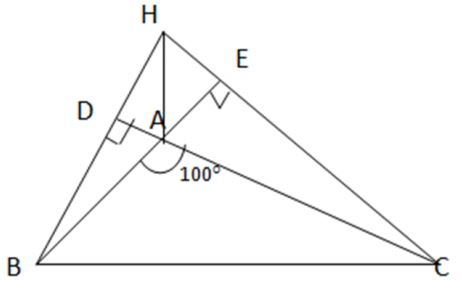Let E be the foot of the altitude from C to AB, and D the foot of the altitude from B to AC

=> HC BE, HB CD

We have \(\widehat{BAC}\) + \(\widehat{BAD}\) = 180°

=> 100° + \(\widehat{BAD}\) = 180°

∆ ADB is a right triangle at D => \(\widehat{BAD}\) + \(\widehat{ABD}\) = 90°

=>\(\widehat{ABD}\) = 90°- 80° = 10°

=> \(\widehat{EBH}\) = 10°

∆ BEH is a right triangle at E => \(\widehat{EBH}\) + \(\widehat{BHE}\) = 90°

=>\(\widehat{BHE}\) = 90°- 10° = 80°

=> \(\widehat{BHC}\) = 80°

–>

— *****

### Solve lesson 9.28, page 81 Math 7 Textbook Connecting knowledge volume 2 – KNTT

Consider point O equidistant from the three vertices of triangle ABC. Prove that if O lies on one side of triangle ABC, then ABC is a right triangle

Detailed instructions for solving problem 9.28

Solution method

Considering OAB we have: \(\widehat{OAB}\) + \(\widehat{OBA}\) + \(\widehat{AOB}\)= 180°

=> measure \(\widehat{BAC}\)

So ∆ ABC is square at A

Detailed explanation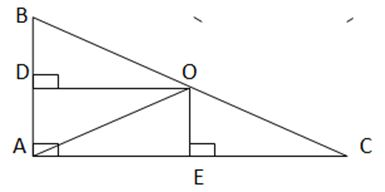O is equidistant from 3 vertices of triangle ABC => O is the intersection of 3 perpendicular bisectors of triangle ABC

=> OA= OB= Oc

=> ∆ OAB is balanced at O. => \(\widehat{OAB}\) = \(\widehat{OBA}\)

∆ OAC is at O ​​=> \(\widehat{OAC}\) + \(\widehat{OCA}\)

Considering OAB we have: \(\widehat{OAB}\) + \(\widehat{OBA}\) + \(\widehat{AOB}\)= 180°

=> 2\(\widehat{OAB}\) + \(\widehat{AOB}\)= 180°

=> \(\widehat{AOB}\)= 180° – 2 \(\widehat{OAB}\)

Similarly we have \(\widehat{AOC}\)= 180° – 2 \(\widehat{OAC}\)

O belongs to BC => \(\widehat{AOB}\) + \(\widehat{AOC}\)= 180°

=.> 180° – 2 \(\widehat{OAB}\) + 180° – 2 \(\widehat{OAC}\) = 180°

=> 360° – 180° = 2 \(\widehat{OAB}\) + 2 \(\widehat{OAC}\)

=> 180° = 2 (\(\widehat{OAB}\) + \(\widehat{OAC}\) )

=> \(\widehat{BAC}\) = 90°

=> ABC is square at A

–>

— *****

### Solve problem 9.29 page 81 Math textbook 7 Connecting knowledge volume 2 – KNTT

a) There is a machine part (outer contour is a circle) broken. (H.9.46). How to determine the radius of this border?

b) On the map, three residential areas planned at points A, B, C are not aligned. Find on the map a point M equidistant from A, B, C to plan a school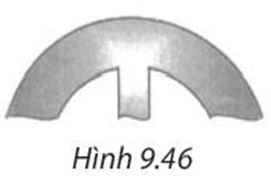Detailed instructions for solving problem 9.29

Solution method

a) Take three distinct points A, B, C

Draw the midline of side AB and side BC

The radius of the circle to be found is the length of the segment OB

Illustrator

b)

Draw the medians of the segments AB, AC, BC

M is the point to be determined

Draw illustrations

Detailed explanation

a)

Take three distinguishing points A, B, C on the outer contour of the machine part.

Draw the midline of side AB and side BC. These two orthogonals intersect at O. Then O is the center to be determined.

The radius of the circle to be found is the length of the segment OB (or OA or OC).

I have an illustration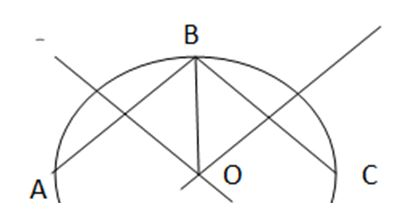b)

Draw the medians of the segments AB, AC, BC

These 3 orthogonal lines intersect at M. Then MA=MB=MC

M is the point to be determined

I have an illustration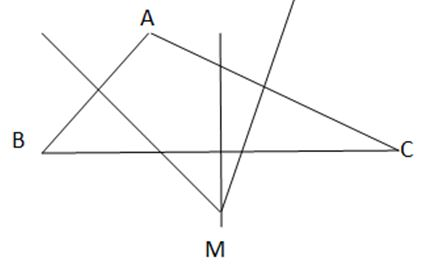–>

— *****

### Solution 9.30 page 81 Math textbook 7 Connecting knowledge volume 2 – KNTT

Let two non-perpendicular lines b and c intersect at point A and give a point H that is not in b and c (H.9.47). Find point B on b and point C on c such that triangle ABC has H as orthocenter.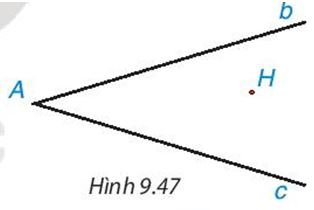Detailed explanation guide

Solution method

Draw HD line c, HE line b

Take point B on line b such that BE lies between B and A, draw a line perpendicular to AH

Detailed explanation

Draw HD line c at point D, HE line b at point E

Connect A to H. Take point B on line b such that BE lies between B and A

From B draw a line perpendicular to AH, that line intersects line c at 1 point. That point is the C point

=> H is the orthocenter of triangle ABC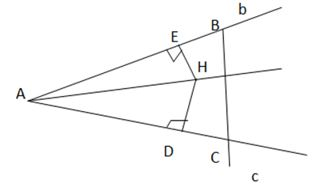–>

— *****